# Area + surface area - math problems

#### Number of problems found: 484

• Base side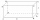In a quadrilateral prism, are known surface area S = 12400 mm2 base side m = 40mm and prism height = 120mm, what is the length of base side n =?
• The surfaceThe surface of the cylinder is 1570 cm2, its height is 15 cm. Find its volume and radius of the base.
• A butterA butter cube with an edge 6.5 cm long is packed in a package with dimensions a = 28 cm, b = 15 cm. Calculate how many cm2 the package is larger than the surface of the cube.
• The conical roof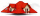The conical roof above the warehouse has a diameter of the lower part (base) d = 11.2 m and a height v = 3.3 m. How many rectangular steel plates with dimensions of 1.4 m and 0.9 m were needed for the production of this roof, if the seams and waste requir
• School modelThe beech school model of a regular quadrilateral pyramid has a base 20 cm long and 24 cm high. Calculate a) the surface of the pyramid in square decimeters, b) the mass of the pyramid in kilograms if the density of the beech is ρ = 0,8 g/cm ^ 3
• Right-angled triangle baseFind the volume and surface area of a triangular prism with a right-angled triangle base if the length of the prism base legs are 7.2 cm and 4.7 cm and the height of a prism is 24 cm.
• How toHow to find a total surface of a rectangular pyramid if each face is to be 8 dm high and the base is 10 dm by 6 dm.
• Empty aquariumHow much does an empty aquarium weigh with dimensions: length = 40 cm, width = 30 cm, height = 20 cm, if 1 dm2 of glass weighs 300 g? Calculate its weight in kilograms.
• Frustrum - volume, areaCalculate the surface and volume of the truncated cone, the radius of the smaller figure is 4 cm, the height of the cone is 4 cm and the side of the truncated cone is 5 cm.
• Frustrum - volume, area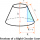Calculate the surface and volume of a truncated rotating cone with base radii of 8 cm and 4 cm, height 5 cm.Find the surface area of a regular quadrilateral pyramid if for its volume V and body height v and the base edge a applies: V = 2.8 m ^ 3, v = 2.1 m
• Regular square prismThe volume of a regular square prism is 192 cm3. The size of its base edge and the body height is 1: 3. Calculate the surface of the prism.
• Cylinder from paperFrom a rectangle measuring 20 x 30 cm, we roll a cylinder with a height of 30 cm. Find its volume and surface.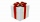How much wrapping paper is needed to wrap a cube-shaped gift with an edge measuring 15 cm?
• Trapezoidal base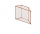Calculate the surface and volume of a quadrilateral prism with a trapezoidal base, where a = 7 cm, b = 4 cm, c = 5 cm, d = 4 cm, height of trapezium v = 3.7 cm and the height of the prism h = 5 cm.
• Axial sectionCalculate the volume and surface of a cone whose axial section is an equilateral triangle with side length a = 18cm.
• The surfaceThe surface of a truncated rotating cone with side s = 13 cm is S = 510π cm2. Find the radii of the bases when their difference in lengths is 10cm.
• The tetrahedron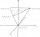Calculate the surface area and volume of a regular tetrahedron 4.9 cm high, the base edge has a length of 6 cm.
• The blockThe block, the edges formed by three consecutive GP members, has a surface area of 112 cm2. The sum of the edges that pass through one vertex is 14 cm. Calculate the volume of this block.
• Cans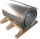How many m2 of metal sheet is needed to produce 20,000 cans in the shape of a cylinder with a base radius and a height of 5 cm.

Do you have an exciting math question or word problem that you can't solve? Ask a question or post a math problem, and we can try to solve it.

We will send a solution to your e-mail address. Solved examples are also published here. Please enter the e-mail correctly and check whether you don't have a full mailbox.

Area - math problems. Examples for the calculation of the surface area of ​​the solid object .